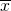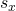F Distribution and One-Way ANOVA

# Lab: One-Way ANOVA

One-Way ANOVA

Class Time:

Names:

Student Learning Outcome
• The student will conduct a simple one-way ANOVA test involving three variables.
Collect the Data
1. Record the price per pound of eight fruits, eight vegetables, and eight breads in your local supermarket.
2. Explain how you could try to collect the data randomly.
Analyze the Data and Conduct a Hypothesis Test
1. State the null hypothesis and the alternative hypothesis.
2. Compute the following:
1. Fruit:
1.= ______
2.= ______
3. n = ______
2. Vegetables:
1.= ______
2.= ______
3. n = ______
1.= ______
2.= ______
3. n = ______
3. Find the following:
1. df(num) = ______
2. df(denom) = ______
4. State the approximate distribution for the test.
5. Test statistic: F = ______
6. Sketch a graph of this situation. CLEARLY, label and scale the horizontal axis and shade the region(s) corresponding to the p-value.
7. p-value = ______
8. Test at α = 0.05. State your decision and conclusion.
1. Decision: Why did you make this decision?
2. Conclusion (write a complete sentence).
3. Based on the results of your study, is there a need to investigate any of the food groups’ prices? Why or why not?